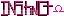-=+=- -=+=- -=+=- -=+=- -=+=- -=+=- -=+=- -=+=- -=+=- -=+=- -=+=- -=+=- -=+=- -=+=- -=+=- -=+=- -=+=- -=+=- -=+=- -=+=- -=+=- -=+=- -=+=- -=+=- -=+=- -=+=- -=+=- -=+=- -=+=- -=+=- (c) WidthPadding Industries 1987 0|73|0 -=+=- -=+=- -=+=- -=+=- -=+=- -=+=- -=+=- -=+=- -=+=- -=+=- -=+=- -=+=- -=+=- -=+=- -=+=- -=+=- -=+=- -=+=- -=+=- -=+=- -=+=- -=+=- -=+=- -=+=- -=+=- -=+=- -=+=- -=+=- -=+=- -=+=-
SoCoder -> Snippet Home -> Website Development

StickyCreated : 04 January 2010

System : Nintendo Wii
Language : Flash

### Integer\\IP converter

Converts between integers and IPs

Just two functions I wrote that convert an IP to an integer or vice versa. Useful for inserting into databases as an int only needs four bytes whereas a text representation of an IP can need up to 15.

This one converts from an integer into an IP.

And this does the reverse, converting an IP to an integer.

Tuesday, 05 January 2010, 00:27
Jayenkai*wonders why you used powers rather than bitshifting.*

Nice and simple.
This is one of those really simple space saving methods that everyone seems to completely forget about.Tuesday, 05 January 2010, 04:09
StickyI used powers instead of bitshifting because I couldn't work out how to force it as an unsigned int - as soon as I bitshifted 24 it made it negative because it was signed. So, powers were just easier in the end.Tuesday, 05 January 2010, 04:20
JayenkaiOh yeah.. hmm.. *shrugs*Wednesday, 06 January 2010, 20:11
SchererererYour comment about negative numbers appearing in your integer inspired me to write a quick article on the way signed numbers are stored in a computer.

www.socoder.net/index.php?article=21718

hope it helps! (particularly the comments on overflow)